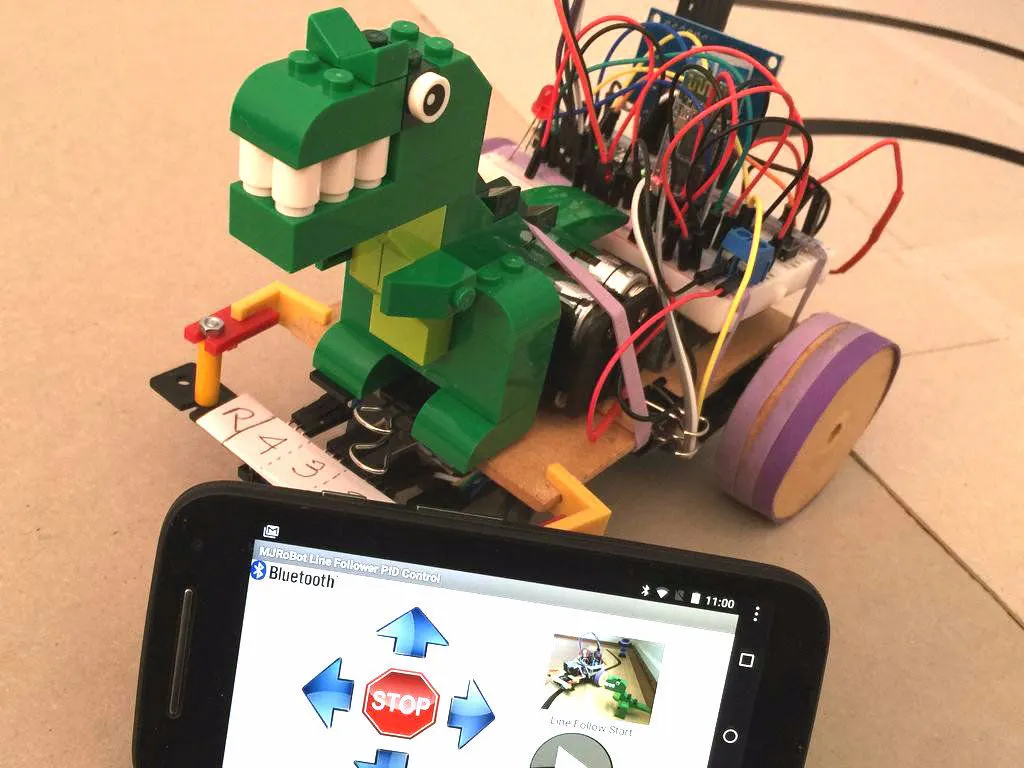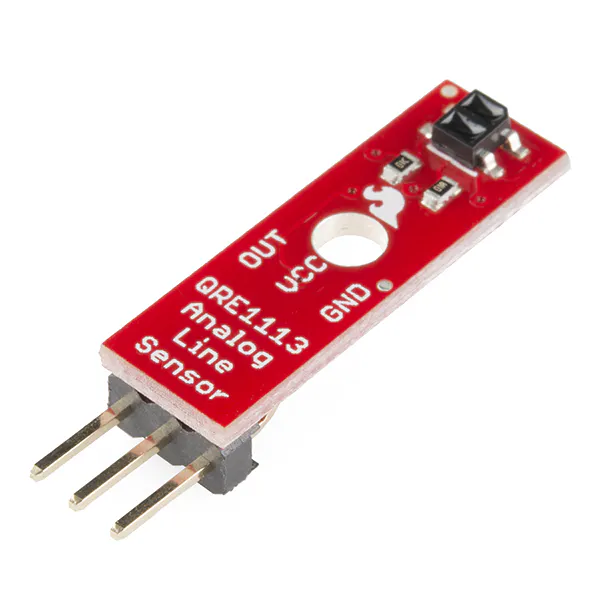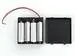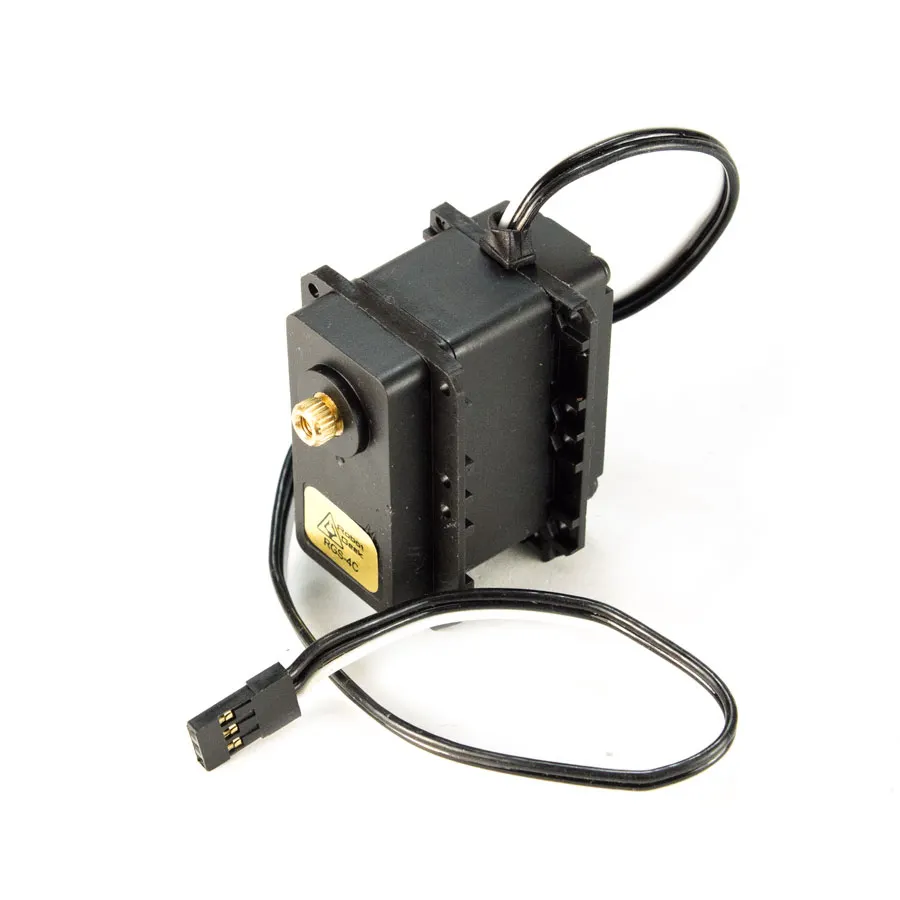# Line Follower Robot - PID Control - Android Setup

Line Follower Robot with PID control, using an Android device to easily setup the main control parameters for better and fast loop tuning.

IntermediateFull instructions provided24 hours39,841## Things used in this project

### Hardware componentsArduino Nano R3
×1SparkFun RedBot Sensor - Line Follower
×1
 TCRT5000 4CH Infrared Line Track Follower Sensor Module
×1
 HC-06 Bluetooth Module
×1Android device
×14xAA battery holder
×2RobotGeek Continuous Rotation Servo
×2

### Software apps and online servicesArduino IDEMIT App Inventor 2

## Code

### Code snippet #1

Plain text
```#include <Servo.h> // Servo library
Servo leftServo;
Servo rightServo;

Void setup()
{
leftServo.attach(5);
rightServo.attach(3);
leftServo.writeMicroseconds(1500);
rightServo.writeMicroseconds(1500);
}

void loop()
{
}
```

### Code snippet #2

Plain text
```while(digitalRead(buttonPin))
{
}
motorTurn (LEFT, 500);
motorTurn (RIGHT, 500);
```

### Code snippet #3

Plain text
```const int lineFollowSensor0 = 12;
const int lineFollowSensor1 = 18;
const int lineFollowSensor2 = 17;
const int lineFollowSensor3 = 16;
const int lineFollowSensor4 = 19;
```

### Code snippet #5

Plain text
```LFSensor = digitalRead(lineFollowSensor0);
```

### Code snippet #6

Plain text
```if((LFSensor== 0 )&&(LFSensor== 0 )&&(LFSensor== 0 )&&(LFSensor== 0 )&&(LFSensor== 1 )) error = 4;

else if((LFSensor== 0 )&&(LFSensor== 0 )&&(LFSensor== 0 )&&(LFSensor== 1 )&&(LFSensor== 1 )) error = 3;

else if((LFSensor== 0 )&&(LFSensor== 0 )&&(LFSensor== 0 )&&(LFSensor== 1 )&&(LFSensor== 0 )) error = 2;

else if((LFSensor== 0 )&&(LFSensor== 0 )&&(LFSensor== 1 )&&(LFSensor== 1 )&&(LFSensor== 0 )) error = 1;

else if((LFSensor== 0 )&&(LFSensor== 0 )&&(LFSensor== 1 )&&(LFSensor== 0 )&&(LFSensor== 0 )) error = 0;

else if((LFSensor== 0 )&&(LFSensor== 1 )&&(LFSensor== 1 )&&(LFSensor== 0 )&&(LFSensor== 0 )) error =- 1;

else if((LFSensor== 0 )&&(LFSensor== 1 )&&(LFSensor== 0 )&&(LFSensor== 0 )&&(LFSensor== 0 )) error = -2;

else if((LFSensor== 1 )&&(LFSensor== 1 )&&(LFSensor== 0 )&&(LFSensor== 0 )&&(LFSensor== 0 )) error = -3;

else if((LFSensor== 1 )&&(LFSensor== 0 )&&(LFSensor== 0 )&&(LFSensor== 0 )&&(LFSensor== 0 )) error = -4;
```

### Code snippet #10

Plain text
```void calculatePID()
{
P = error;
I = I + error;
D = error-previousError;
PIDvalue = (Kp*P) + (Ki*I) + (Kd*D);
previousError = error;
}
```

### Code snippet #11

Plain text
```void motorPIDcontrol()
{
int leftMotorSpeed = 1500 - iniMotorPower - PIDvalue;
int rightMotorSpeed = 1500 + iniMotorPower - PIDvalue;

leftServo.writeMicroseconds(leftMotorSpeed);
rightServo.writeMicroseconds(rightMotorSpeed);
}
```

### Code snippet #12

Plain text
```void loop ()
{
readLFSsensors(); // read sensors, storage values at Sensor Array and calculate "error"
calculatePID();
motorPIDcontrol();
}
```

### Code snippet #15

Plain text
```void loop()
{
switch (mode)
{
case STOPPED:
motorStop();
break;
case NO_LINE:
motorStop();
motorTurn(LEFT, 180);
break;
case FOLLOWING_LINE:
calculatePID();
motorPIDcontrol();
break;
}
}
```

### Code snippet #17

Plain text
```  while (digitalRead(buttonPin) && !mode)
{
checkBTcmd();  // verify if a comand is received from BT remote control
manualCmd ();
command = "";
}
checkPIDvalues();
mode = STOPPED;
```

### Code snippet #18

Plain text
```void manualCmd()
{
switch (command)
{
case 'g':
mode = FOLLOWING_LINE;
break;

case 's':
motorStop(); //turn off both motors
break;

case 'f':
motorForward();
break;

case 'r':
motorTurn(RIGHT, 30);
motorStop();

break;

case 'l':
motorTurn(LEFT, 30);
motorStop();
break;

case 'b':
motorBackward();
break;

case 'p':
Kp = command;
break;

case 'i':
Ki = command;
break;

case 'd':
Kd = command;
break;
}
}
```

### Github

https://github.com/Mjrovai/MJRoBot-Line-Follower

## Credits

### MJRoBot

34 projects • 649 followers
Engineer, MBA, Master in Data Science. Write about Data Science and Electronics with a focus on Physical Computing, IoT, and Robotics.